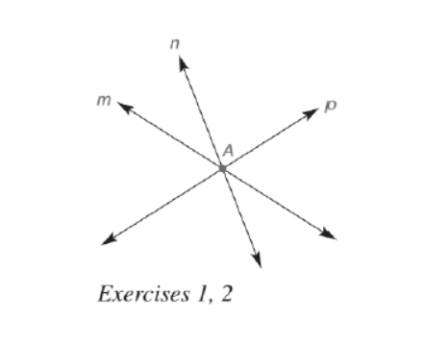Chapter 7.2, Problem 1EElementary Geometry For College St...

7th Edition
Alexander + 2 others
ISBN: 9781337614085

Solutions

Chapter
SectionElementary Geometry For College St...

7th Edition
Alexander + 2 others
ISBN: 9781337614085
Textbook Problem

Note: Exercise preceded by an asterisk are of a more challenging nature.a) In the figure, are lines m, n, and p concurrent?b) If one exists, name the point of concurrence for lines m, n, and p.(a)

To determine

To find:

In the figure, are lines m, n, and p concurrent.

Explanation

Definition:

Concurrent:

A number of lines are concurrent if they have exactly one point in common.

Two or more lines in a plane that have a single point in common are known as concurrent lines. When exactly two lines have a point in common, we generally describe them as intersecting lines rather than concurrent lines.

Given:

(b)

To determine

To find:

If one exists, name the point of concurrence for lines m, n, and p.

Still sussing out bartleby?

Check out a sample textbook solution.

See a sample solution

The Solution to Your Study Problems

Bartleby provides explanations to thousands of textbook problems written by our experts, many with advanced degrees!

Get Started

Evaluate the expression sin Exercises 116. 2322

Finite Mathematics and Applied Calculus (MindTap Course List)

Differentiate. y=sint1+tant

Single Variable Calculus: Early Transcendentals

True or False: n=21(lnn)n converges.

Study Guide for Stewart's Single Variable Calculus: Early Transcendentals, 8th

Using for |x| < 1 and differentiation, find a power series for .

Study Guide for Stewart's Multivariable Calculus, 8th